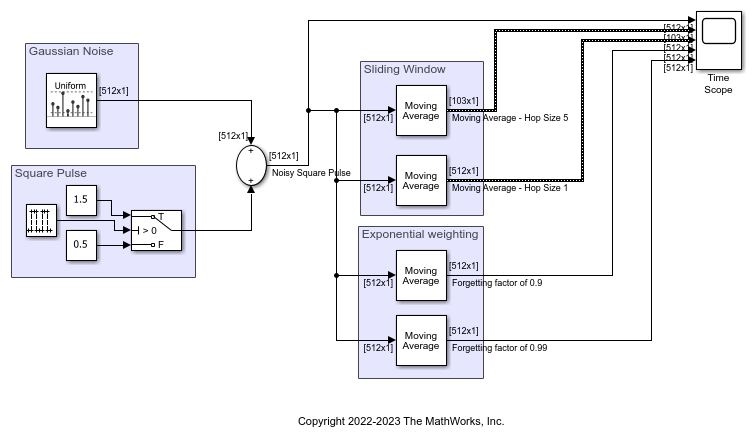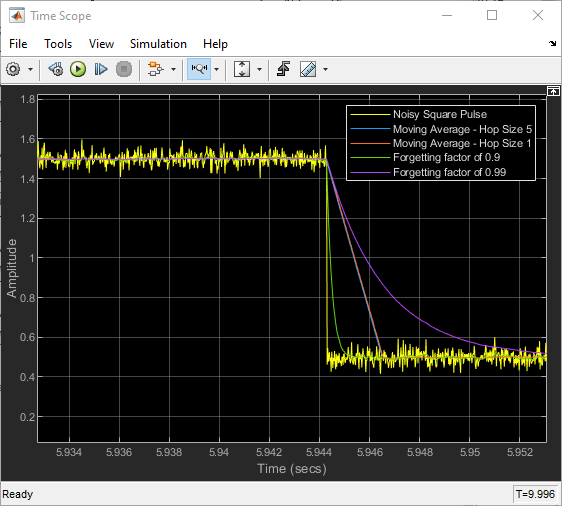# Compute Moving Average of Noisy Step Signal

Compute the moving average of a noisy square wave signal using the Moving Average block. Use the sliding window method with a hop sizeof 5 and 1, and the exponential weighting method with a forgetting factor of 0.9 and 0.99. Compare the output of these two methods. For more details on these methods, see Sliding Window Method and Exponential Weighting Method.

### Open and Run the Model

Open and run the `MovAvgModel.slx` model.

The Pulse Generator (Simulink) block generates a square pulse input signal of 512 samples in width. The Switch (Simulink) block varies the amplitude of the signal between 1.5 and 0.5. Add zero-mean white Gaussian noise with a variance of 0.001 to the square pulse. The frame length of the input signal is 512 samples.

Compute the moving average of this signal across each frame using four Moving Average blocks. In the first two Moving Average blocks that use the sliding window method, the window length is set to 100 and the overlap length is set to 95 and 99, respectively, resulting in a hop size of 5 and 1. In the last two Moving Average blocks that use the exponential weighting method, the forgetting factor is set to 0.9 and 0.99, respectively. View and compare the outputs of these blocks with the original signal in the Time Scope.### Compare Moving Average Outputs

In the sliding window Moving Average blocks, when you select the Allow arbitrary frame length for fixed-size input signals parameter, the dimensions of the moving average output change based on the hop size. If the input is, then the output has an upper bound size of. For more details, see Moving Average.

The last two blocks use the exponential weighting method. In the exponential weighting method, when the signal changes rapidly as in this example, use a lower forgetting factor. When the forgetting factor is low, past data has a lower impact on the current average. This makes the transient sharper even though the output contains more noise. You can see this behavior in the Time Scope when you compare the outputs of the Moving Average blocks for the forgetting factors 0.9 and 0.99.